# Chapter 7 Annotations

Displaying just your data usually isn’t enough – there’s all sorts of other information that can help the viewer interpret the data. In addition to the standard repertoire of axis labels, tick marks, and legends, you can also add individual graphical or text elements to your plot. These elements can be used to add extra contextual information, highlight an area of the plot, or add some descriptive text about the data.

## 7.1 Adding Text Annotations

### 7.1.1 Problem

You want to add a text annotation to a plot.

### 7.1.2 Solution

Use `annotate()` and a text geom (Figure 7.1):

``````p <- ggplot(faithful, aes(x = eruptions, y = waiting)) +
geom_point()

p +
annotate("text", x = 3, y = 48, label = "Group 1") +
annotate("text", x = 4.5, y = 66, label = "Group 2")``````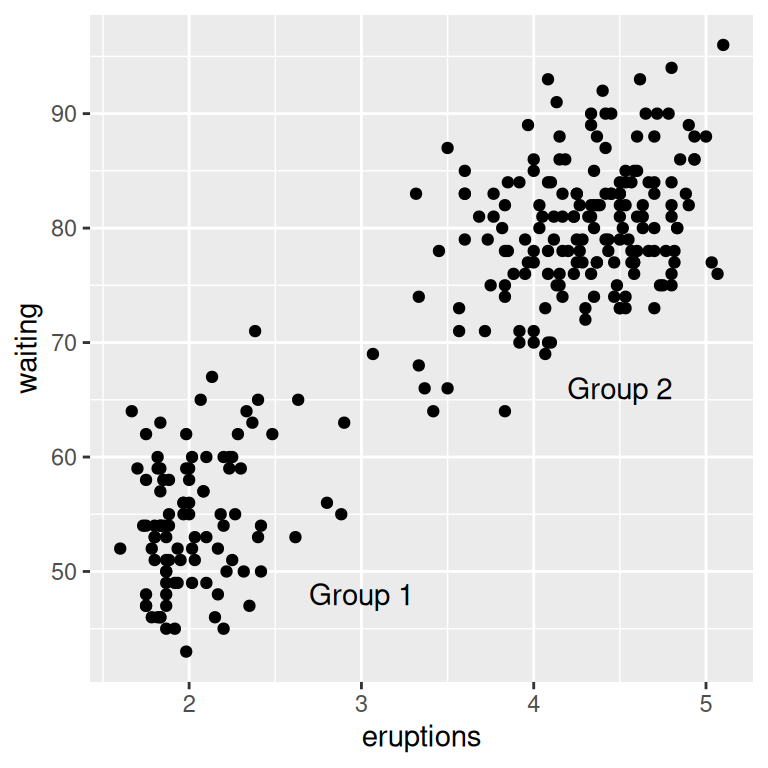Figure 7.1: Text annotations

### 7.1.3 Discussion

The `annotate()` function can be used to add any type of geometric object. In this case, we used `geom = "text"`.

Other text properties can be specified, as shown in Figure 7.2:

``````p +
annotate("text", x = 3, y = 48, label = "Group 1",
family = "serif", fontface = "italic", colour = "darkred", size = 3) +
annotate("text", x = 4.5, y = 66, label = "Group 2",
family = "serif", fontface = "italic", colour = "darkred", size = 3)``````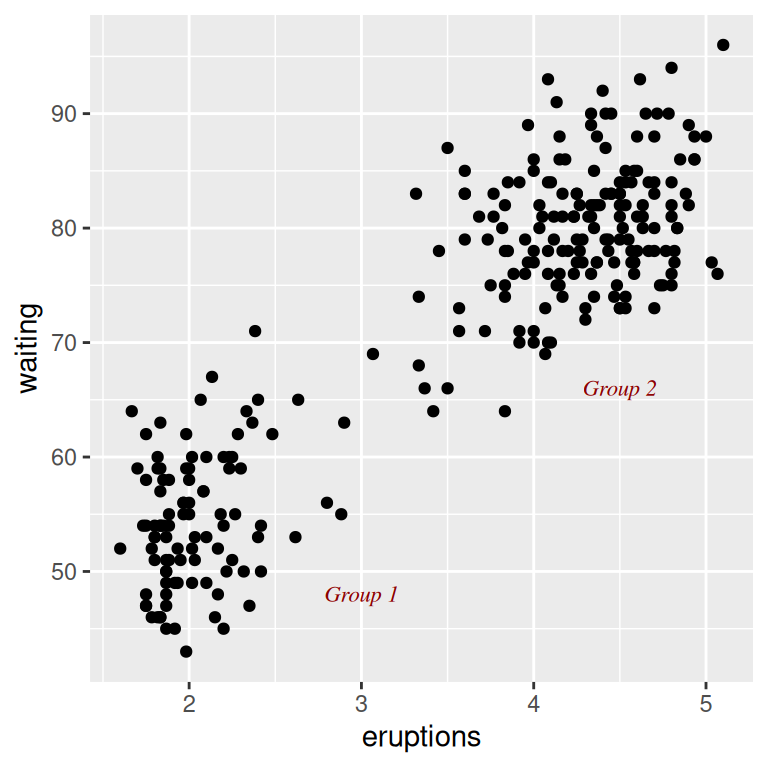Figure 7.2: Modified text properties

Be careful not to use `geom_text()` when you want to add individual text objects. While `annotate(geom = "text")` will add a single text object to the plot, `geom_text()` will create many text objects based on the data, as discussed in Recipe 5.11.

If you use `geom_text()`, the text will be heavily overplotted on the same location, with one copy per data point:

``````p +
# Normal
annotate("text", x = 3, y = 48, label = "Group 1", alpha = .1) +
# Overplotted
geom_text(x = 4.5, y = 66, label = "Group 2", alpha = .1)``````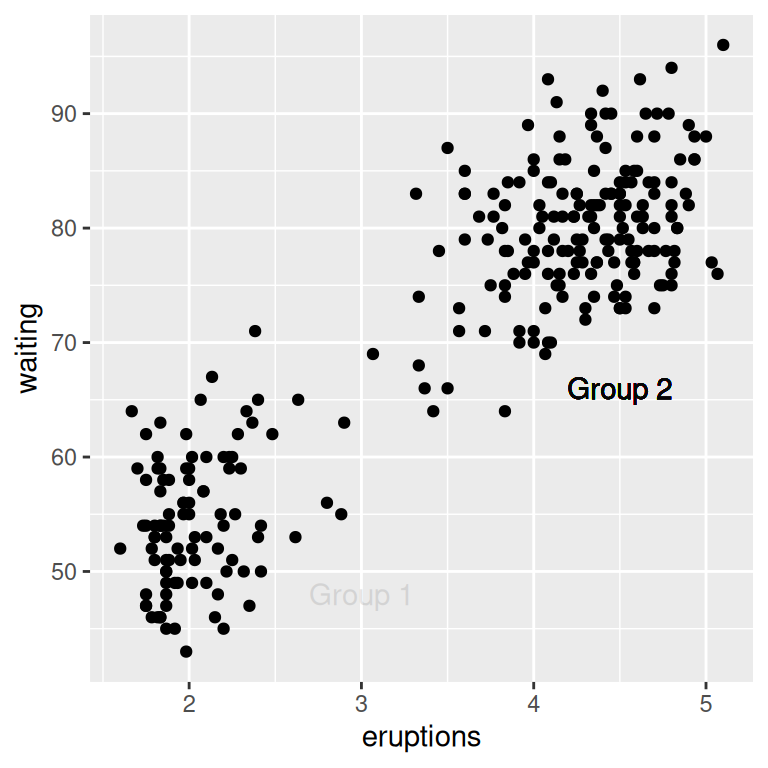Figure 7.3: Overplotting one of the labels – both should be 90% transparent

In Figure 7.3, each text label is 90% transparent, making it clear which one is overplotted. The overplotting can lead to output with aliased (jagged) edges when outputting to a bitmap.

If the axes are continuous, you can use the special values `Inf` and `-Inf` to place text annotations at the edge of the plotting area, as shown in Figure 7.4. You will also need to adjust the position of the text relative to the corner using `hjust` and `vjust` – if you leave them at their default values, the text will be centered on the edge. It may take a little experimentation with these values to get the text positioned to your liking:

``````p +
annotate("text", x = -Inf, y = Inf, label = "Upper left", hjust = -.2, vjust = 2) +
annotate("text", x = mean(range(faithful\$eruptions)), y = -Inf, vjust = -0.4,
label = "Bottom middle")``````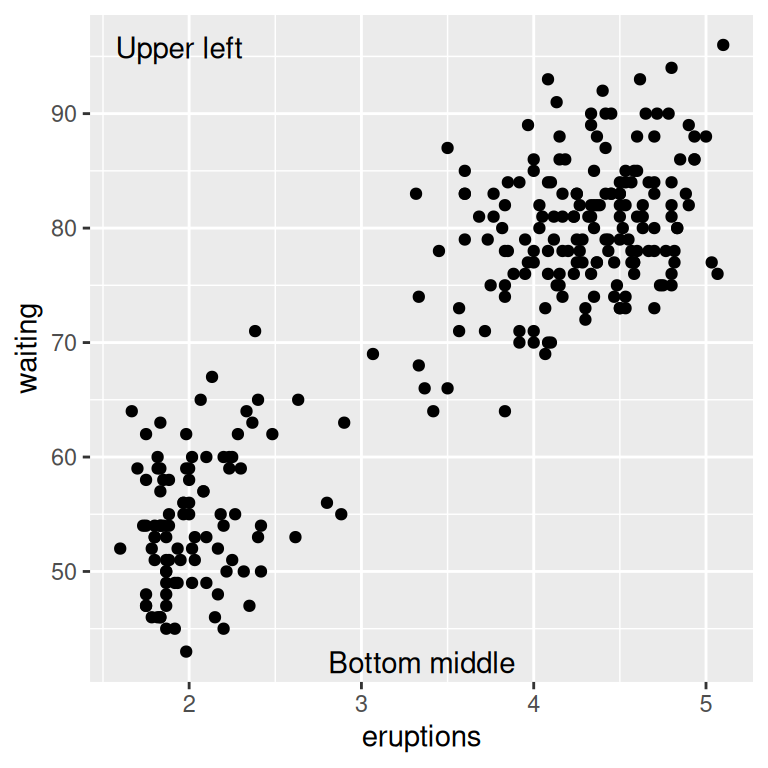Figure 7.4: Text positioned at the edge of the plotting area

See Recipe 5.11 for making a scatter plot with text.

For more on controlling the appearance of the text, see Recipe 9.2.

## 7.2 Using Mathematical Expressions in Annotations

### 7.2.1 Problem

You want to add a text annotation with mathematical notation.

### 7.2.2 Solution

Use `annotate(geom = "text")` with `parse = TRUE` (Figure 7.5):

``````# A normal curve
p <- ggplot(data.frame(x = c(-3,3)), aes(x = x)) +
stat_function(fun = dnorm)

p +
annotate("text", x = 2, y = 0.3, parse = TRUE,
label = "frac(1, sqrt(2 * pi)) * e ^ {-x^2 / 2}")``````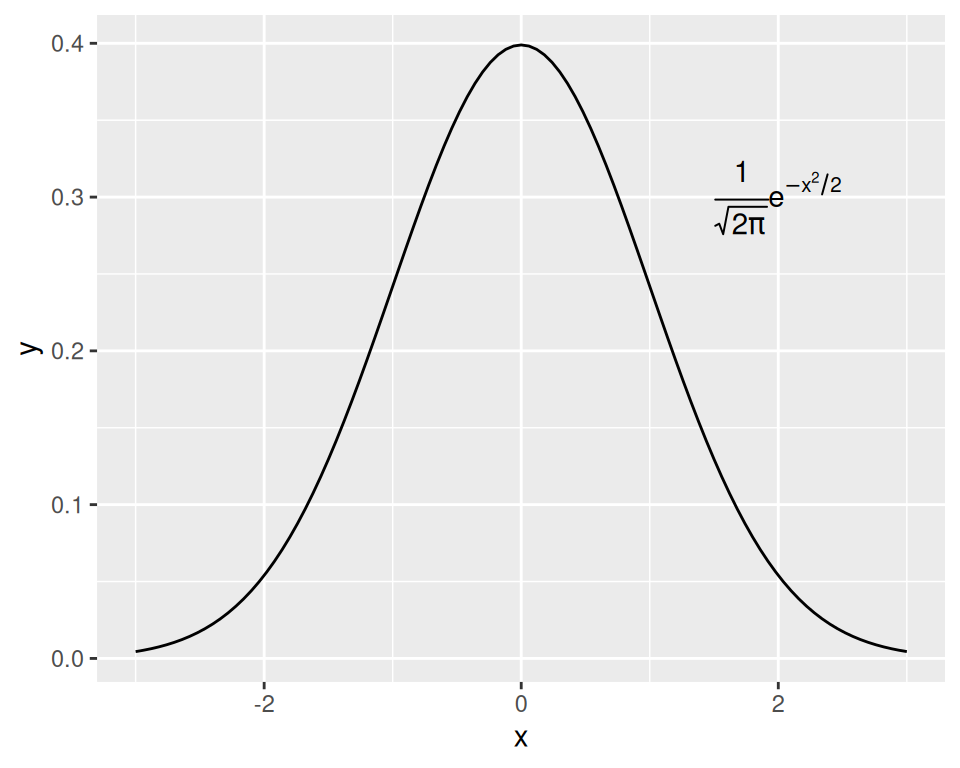Figure 7.5: Annotation with mathematical expressions

### 7.2.3 Discussion

Mathematical expressions made with text geoms using `parse = TRUE` in ggplot2 have a format similar to those made with `plotmath` and `expression` in base R, except that they are stored as strings, rather than as expression objects.

To mix regular text with expressions, use single quotes within double quotes (or vice versa) to mark the plain-text parts. Each block of text enclosed by the inner quotes is treated as a variable in a mathematical expression. Bear in mind that, in R’s syntax for mathematical expressions, you can’t simply put a variable right next to another without something else in between. To display two variables next to each other, as in Figure 7.6, put a `*` operator between them; when displayed in a graphic, this is treated as an invisible multiplication sign (for a visible multiplication sign, use `%*%`):

``````p +
annotate("text", x = 0, y = 0.05, parse = TRUE, size = 4,
label = "'Function:  ' * y==frac(1, sqrt(2*pi)) * e^{-x^2/2}")``````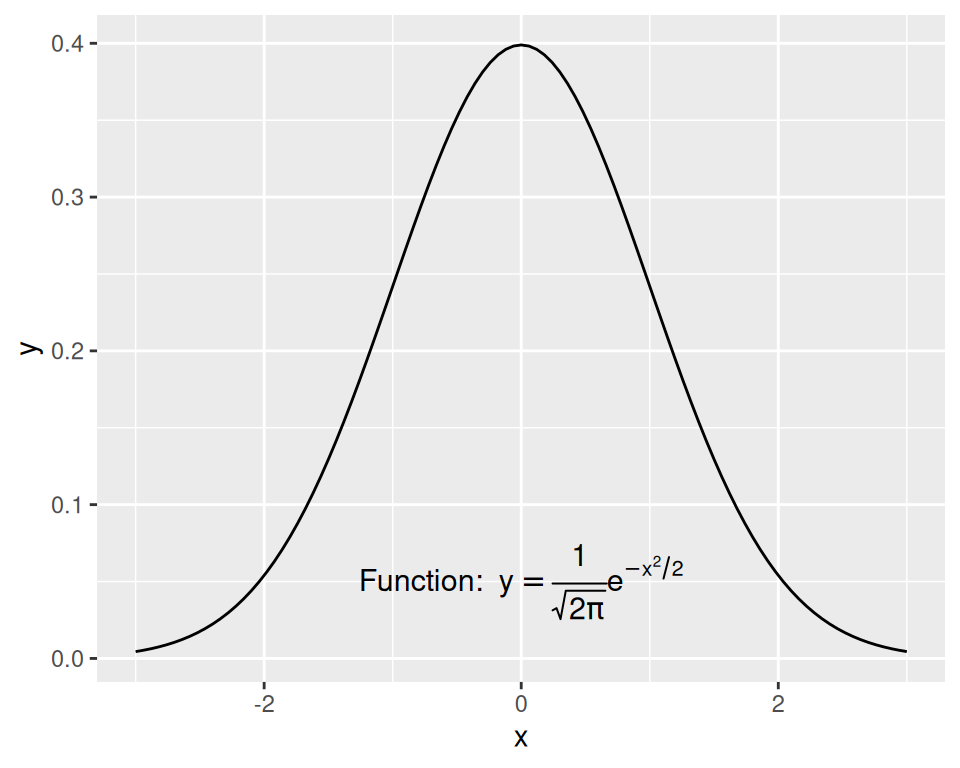Figure 7.6: Mathematical expression with regular text

See `?plotmath` for many examples of mathematical expressions, and `?demo(plotmath)` for graphical examples of mathematical expressions.

See Recipe 5.9 for adding regression coefficients to a graph.

For using other fonts in mathematical expressions, see Recipe 14.6.

## 7.3 Adding Lines

### 7.3.1 Problem

You want to add lines to a plot.

### 7.3.2 Solution

For horizontal and vertical lines, use `geom_hline()` and `geom_vline()`, and for angled lines, use `geom_abline()` (Figure 7.7). For this example, we’ll use the `heightweight` data set:

``````library(gcookbook)  # Load gcookbook for the heightweight data set

hw_plot <- ggplot(heightweight, aes(x = ageYear, y = heightIn, colour = sex)) +
geom_point()

# Add horizontal and vertical lines
hw_plot +
geom_hline(yintercept = 60) +
geom_vline(xintercept = 14)

# Add angled line
hw_plot +
geom_abline(intercept = 37.4, slope = 1.75)``````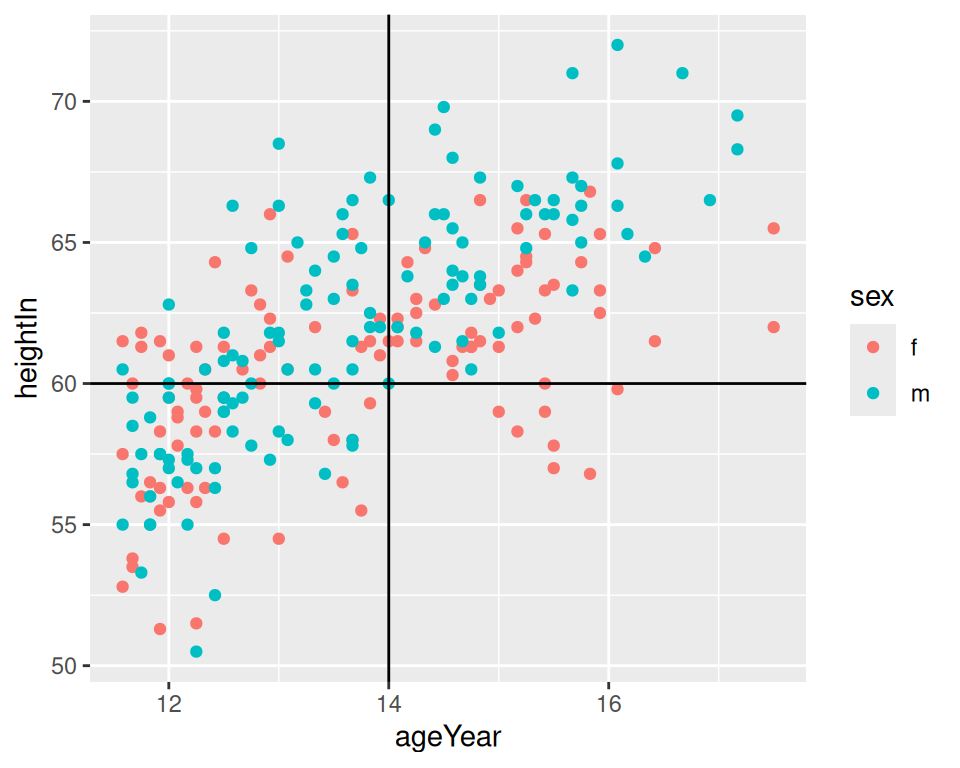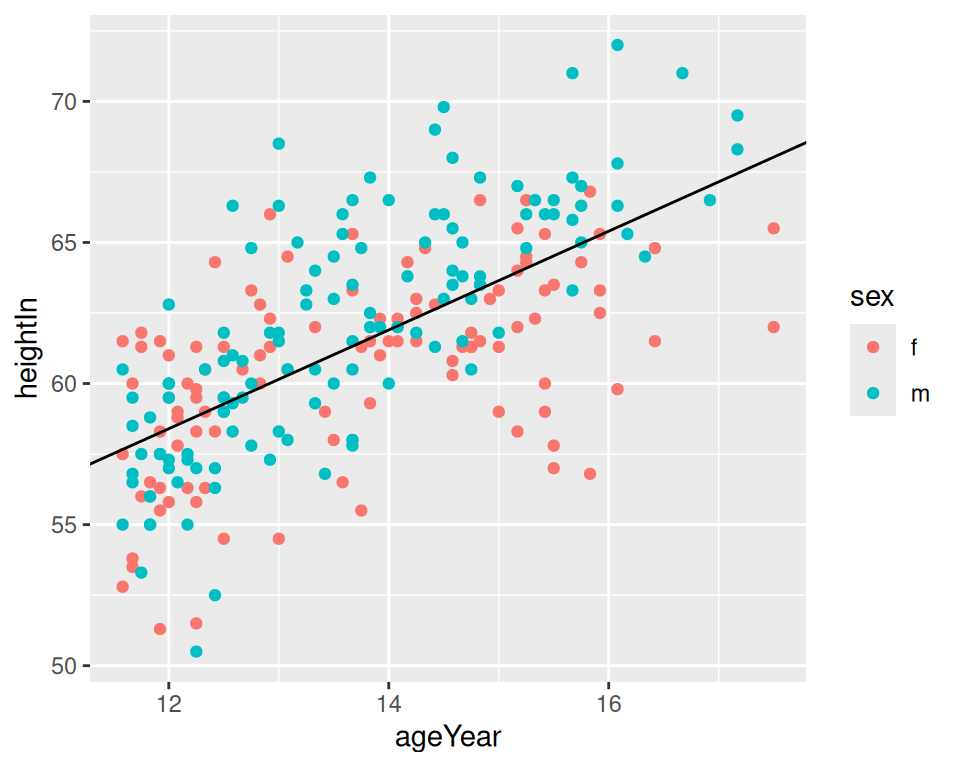Figure 7.7: horizontal and vertical lines (left); angled line (right)

### 7.3.3 Discussion

The previous examples demonstrate setting the positions of the lines manually, resulting in one line drawn for each geom added. It is also possible to map values from the data to `xintercept`, `yintercept`, and so on, and even draw them from another data frame.

Here we’ll take the average height for males and females and store it in a data frame, `hw_means`. Then we’ll draw a horizontal line for each, and set the `linetype` and `size` (Figure 7.8):

``````library(dplyr)

hw_means <- heightweight %>%
group_by(sex) %>%
summarise(heightIn = mean(heightIn))

hw_means
#> # A tibble: 2 x 2
#>   sex   heightIn
#>   <fct>    <dbl>
#> 1 f         60.5
#> 2 m         62.1

hw_plot +
geom_hline(
data = hw_means,
aes(yintercept = heightIn, colour = sex),
linetype = "dashed",
size = 1
)``````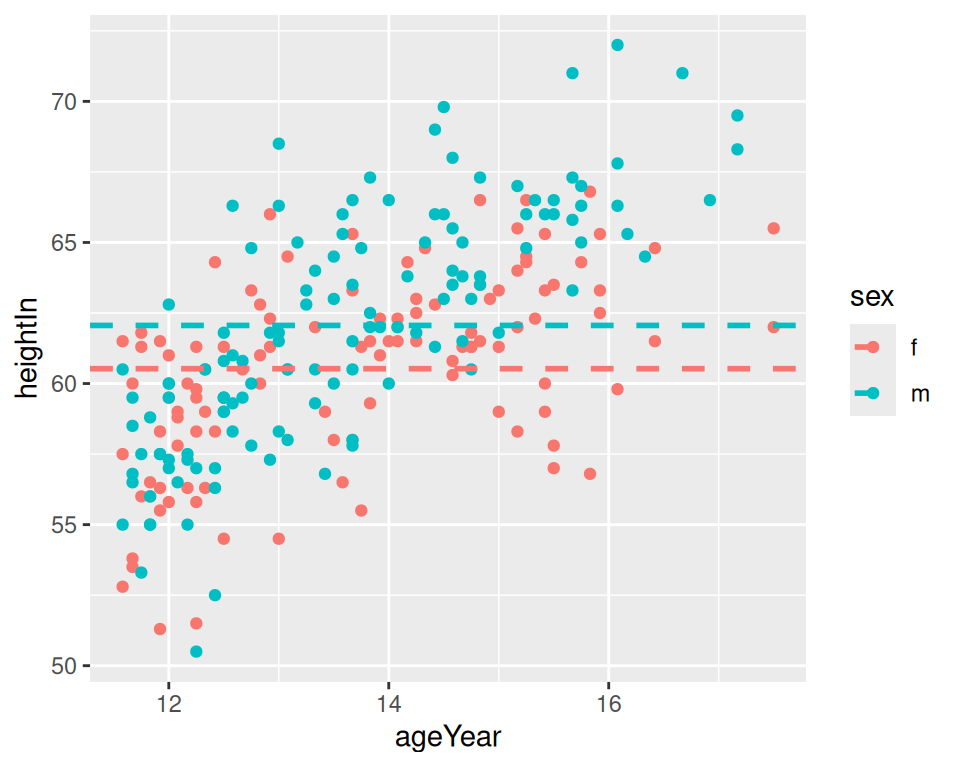Figure 7.8: Multiple lines, drawn at the mean of each group

If one of the axes is discrete rather than continuous, you can’t specify the intercepts as just a character string – they must still be specified as numbers. If the axis represents a factor, the first level has a numeric value of 1, the second level has a value of 2, and so on. You can specify the numerical intercept manually, or calculate the numerical value using `which(levels(...))` (Figure 7.9):

``````pg_plot <- ggplot(PlantGrowth, aes(x = group, y = weight)) +
geom_point()

pg_plot +
geom_vline(xintercept = 2)

pg_plot +
geom_vline(xintercept = which(levels(PlantGrowth\$group) == "ctrl"))``````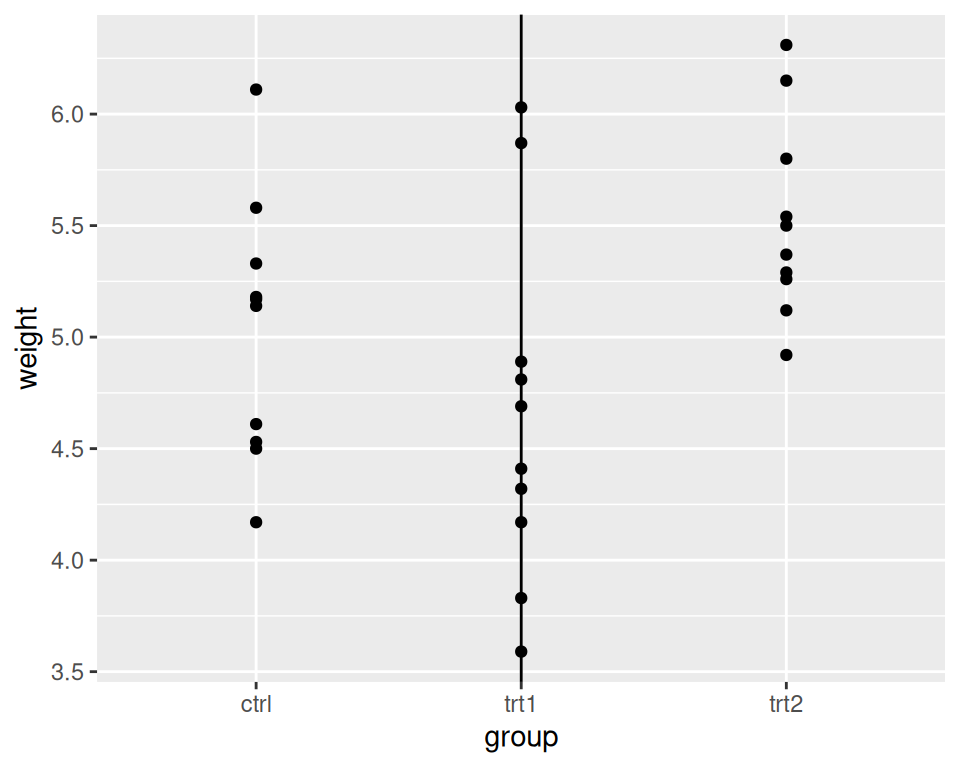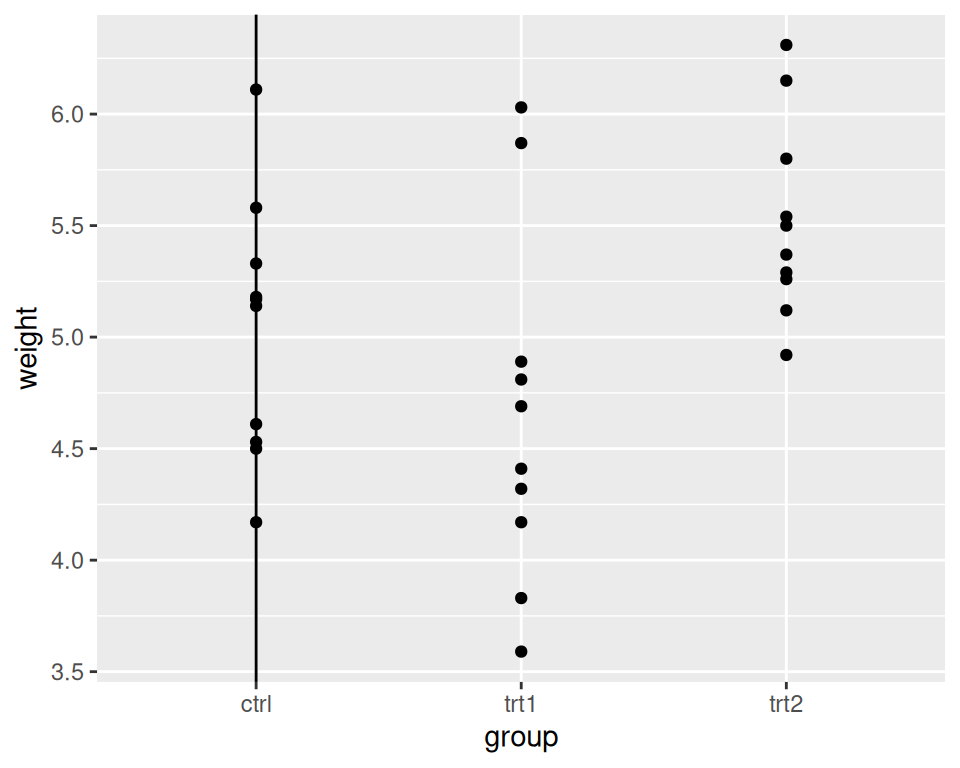Figure 7.9: Lines with a discrete axis

Note

You may have noticed that adding lines differs from adding other annotations. Instead of using the `annotate()` function, we’ve used `geom_hline()` and friends. This is because old versions of ggplot2 didn’t have the `annotate()` function. The line geoms had code to handle the special cases where they were used to add a single line, and changing it would break backward compatibility.

For adding regression lines, see Recipes Recipe 5.6 and Recipe 5.7.

Lines are often used to indicate summarized information about data. See Recipe 15.17 for more on how to summarize data by groups.

## 7.4 Adding Line Segments and Arrows

### 7.4.1 Problem

You want to add line segments or arrows to a plot.

### 7.4.2 Solution

Use `annotate("segment")`. In this example, we’ll use the climate data set and use a subset of data from the Berkeley source (Figure 7.10):

``````library(gcookbook) # Load gcookbook for the climate data set

p <- ggplot(filter(climate, Source == "Berkeley"), aes(x = Year, y = Anomaly10y)) +
geom_line()

p +
annotate("segment", x = 1950, xend = 1980, y = -.25, yend = -.25)``````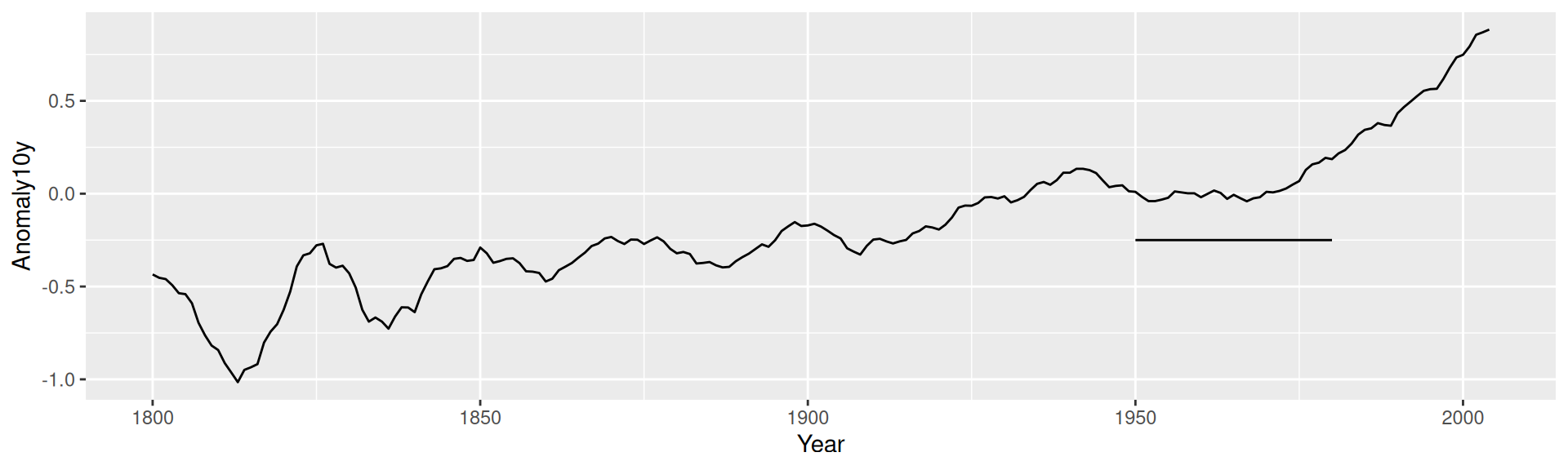Figure 7.10: Line segment annotation

### 7.4.3 Discussion

It’s possible to add arrowheads or flat ends to the line segments, using `arrow()` from the grid package. In this example, we’ll do both (Figure 7.11):

``````library(grid)
p +
annotate("segment", x = 1850, xend = 1820, y = -.8, yend = -.95,
colour = "blue", size = 2, arrow = arrow()) +
annotate("segment", x = 1950, xend = 1980, y = -.25, yend = -.25,
arrow = arrow(ends = "both", angle = 90, length = unit(.2,"cm")))``````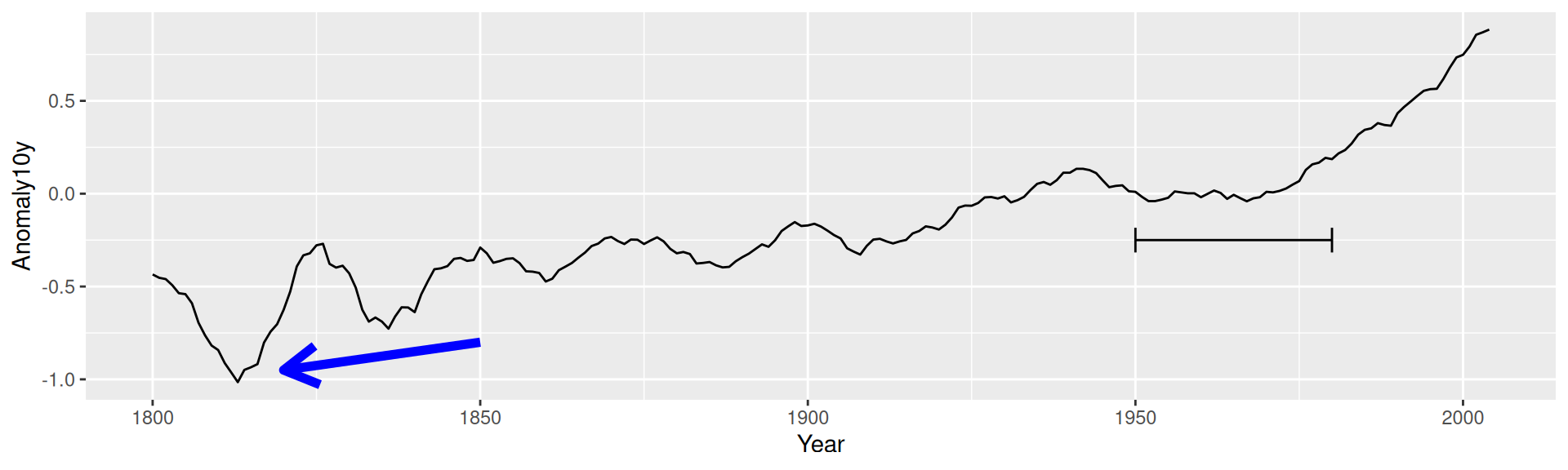Figure 7.11: Line segments with arrow heads

The default angle is 30, and the default length of the arrowhead lines is 0.2 inches.

If one or both axes are discrete, the x and y positions are such that the categorical items have coordinate values 1, 2, 3, and so on.

For more information about the parameters for drawing arrows, load the grid package and see `?arrow`.

### 7.5.1 Problem

You want to add a shaded region.

### 7.5.2 Solution

Use `annotate("rect")` (Figure 7.12):

``````library(gcookbook) # Load gcookbook for the climate data set

p <- ggplot(filter(climate, Source == "Berkeley"), aes(x = Year, y = Anomaly10y)) +
geom_line()

p +
annotate("rect", xmin = 1950, xmax = 1980, ymin = -1, ymax = 1,
alpha = .1,fill = "blue")``````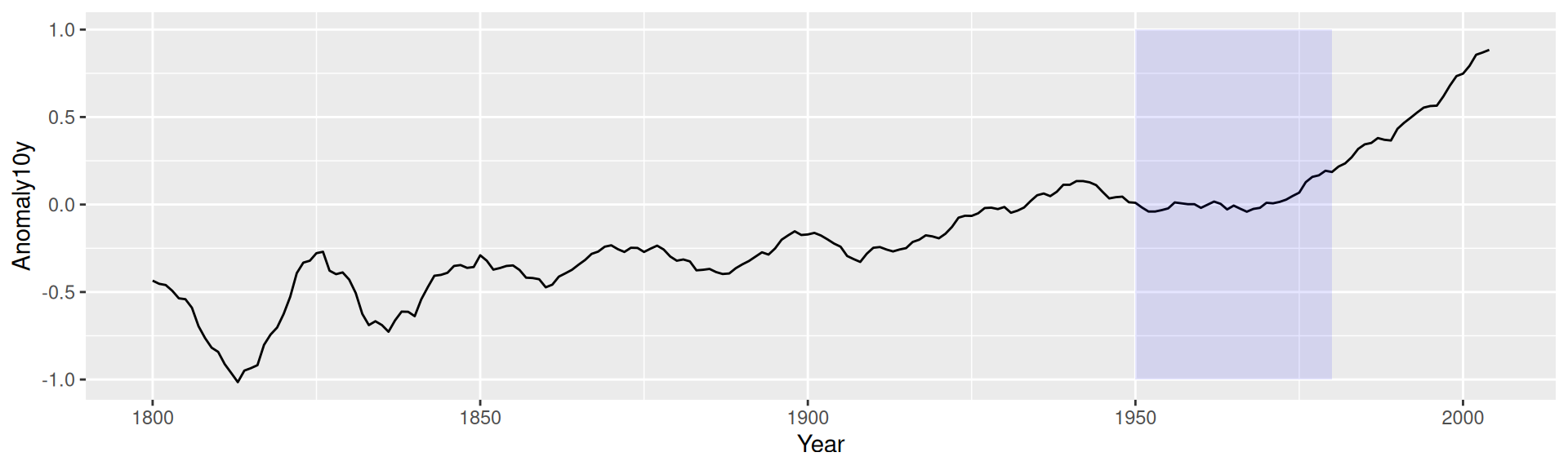Figure 7.12: A shaded rectangle

### 7.5.3 Discussion

Each layer is drawn in the order that it’s added to the ggplot object, so in the preceding example, the rectangle is drawn on top of the line. It’s not a problem in that case, but if you’d like to have the line above the rectangle, add the rectangle first, and then the line.

Any geom can be used with `annotate()`, as long as you pass in the proper parameters. In this case, `geom_rect()` requires min and max values for x and y.

## 7.6 Highlighting an Item

### 7.6.1 Problem

You want to change the color of an item to make it stand out.

### 7.6.2 Solution

To highlight one or more items, create a new column in the data and map it to the color. In this example, we’ll create a copy of the PlantGrowth data set called `pg_mod` and create a new column, `hl`, which is set to `no` if the case was in the control group or treatment 1 group, and set to `yes` if the case was in the treatment 2 group:

``````library(dplyr)

pg_mod <- PlantGrowth %>%
mutate(hl = recode(group, "ctrl" = "no", "trt1" = "no", "trt2" = "yes"))``````

Then we’ll plot this data with specified colors, and hiding the legend (Figure 7.13):

``````ggplot(pg_mod, aes(x = group, y = weight, fill = hl)) +
geom_boxplot() +
scale_fill_manual(values = c("grey85", "#FFDDCC"), guide = FALSE)``````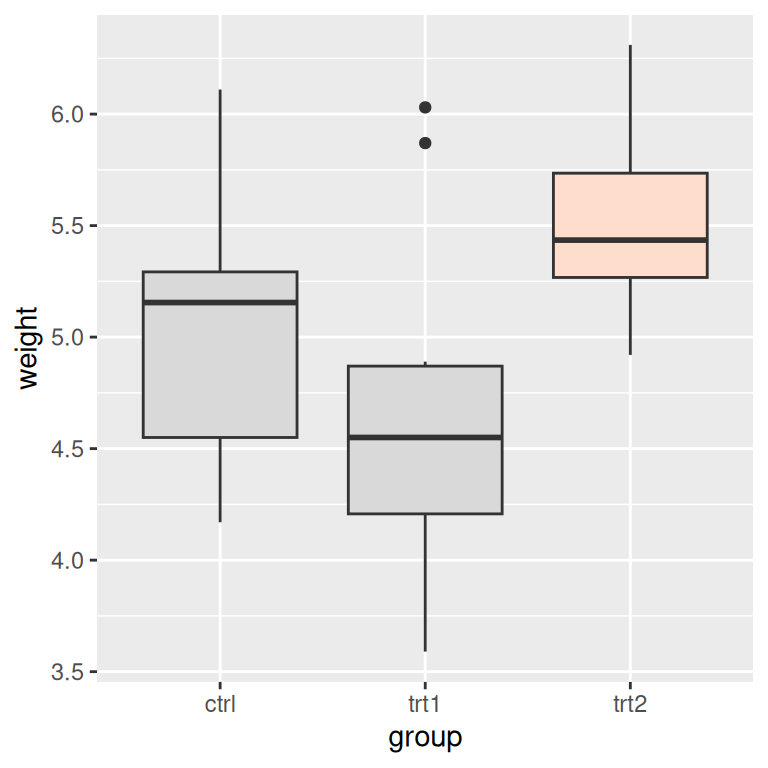Figure 7.13: Highlighting one item

### 7.6.3 Discussion

If you have a small number of items, as in this example, instead of creating a new column you could use the original one and specify the colors for every level of that variable. For example, the following code will use the group column from `PlantGrowth` and manually set the colors for each of the three levels. The result will appear the same as with the preceding code:

``````ggplot(PlantGrowth, aes(x = group, y = weight, fill = group)) +
geom_boxplot() +
scale_fill_manual(values = c("grey85", "grey85", "#FFDDCC"), guide = FALSE)``````

For more information about removing the legend, see Recipe 10.1.

## 7.7 Adding Error Bars

### 7.7.1 Problem

You want to add error bars to a graph.

### 7.7.2 Solution

Use `geom_errorbar()` and map variables to the values for `ymin` and `ymax`. Adding the error bars is done the same way for bar graphs and line graphs, as shown in Figure 7.14 (notice that default y range is different for bars and lines, though):

``````library(gcookbook) # Load gcookbook for the cabbage_exp data set
library(dplyr)

# Take a subset of the cabbage_exp data for this example
ce_mod <- cabbage_exp %>%
filter(Cultivar == "c39")

# With a bar graph
ggplot(ce_mod, aes(x = Date, y = Weight)) +
geom_col(fill = "white", colour = "black") +
geom_errorbar(aes(ymin = Weight - se, ymax = Weight + se), width = .2)

# With a line graph
ggplot(ce_mod, aes(x = Date, y = Weight)) +
geom_line(aes(group = 1)) +
geom_point(size = 4) +
geom_errorbar(aes(ymin = Weight - se, ymax = Weight + se), width = .2)``````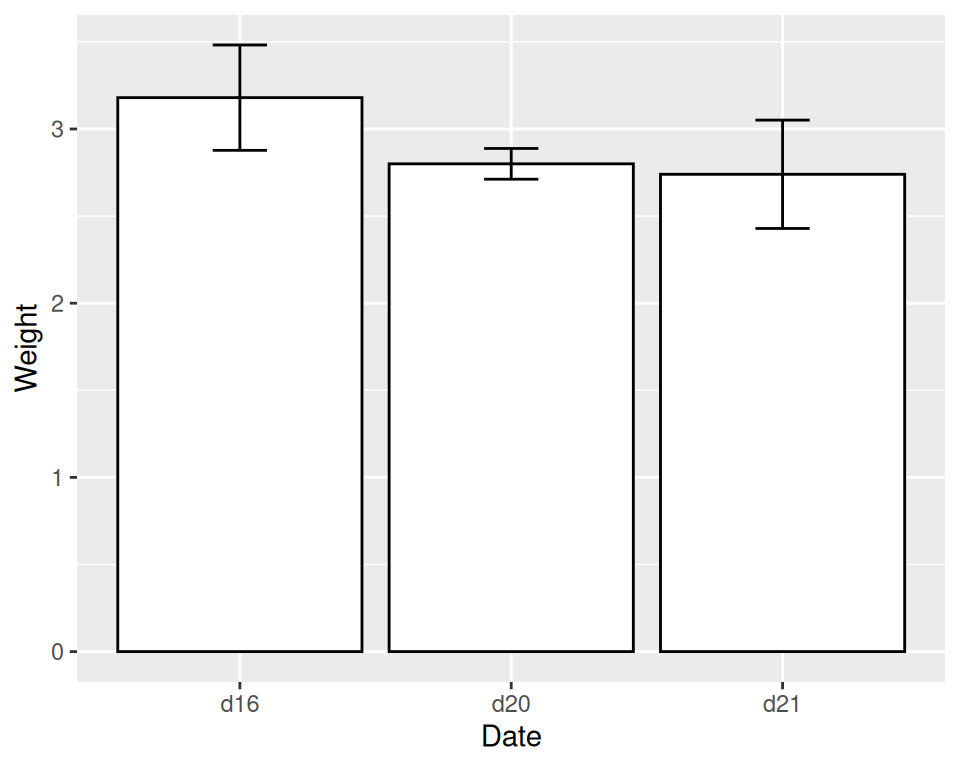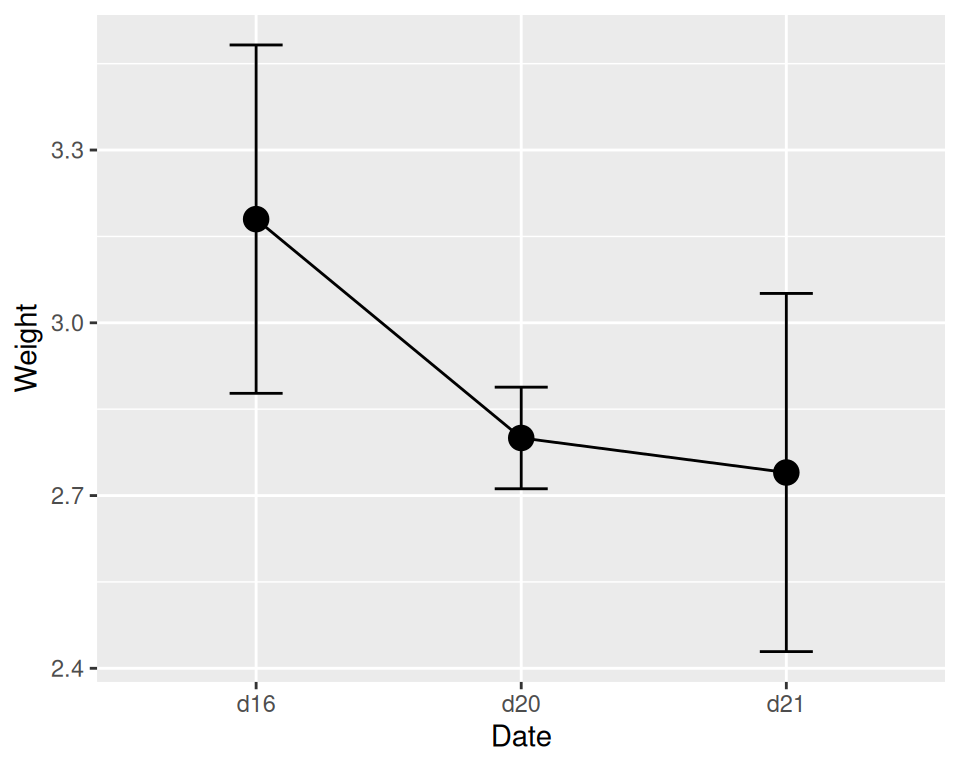Figure 7.14: error bars on a bar graph (left); on a line graph (right)

### 7.7.3 Discussion

In this example, the data already has values for the standard error of the mean (`se`), which we’ll use for the error bars (it also has values for the standard deviation, `sd`, but we’re not using that here):

``````ce_mod
#>   Cultivar Date Weight        sd  n         se
#> 1      c39  d16   3.18 0.9566144 10 0.30250803
#> 2      c39  d20   2.80 0.2788867 10 0.08819171
#> 3      c39  d21   2.74 0.9834181 10 0.31098410``````

To get the values for `ymax` and `ymin`, we took the y variable, `Weight`, and added/subtracted `se`.

We also specified the width of the ends of the error bars, with `width = .2`. It’s best to play around with this to find a value that looks good. If you don’t set the width, the error bars will be very wide, spanning all the space between items on the x-axis.

For a bar graph with groups of bars, the error bars must also be dodged; otherwise, they’ll have the exact same x coordinate and won’t line up with the bars. (See Recipe 3.2 for more information about grouped bars and dodging.)

We’ll work with the full `cabbage_exp` data set this time:

``````cabbage_exp
#>   Cultivar Date Weight        sd  n         se
#> 1      c39  d16   3.18 0.9566144 10 0.30250803
#> 2      c39  d20   2.80 0.2788867 10 0.08819171
#> 3      c39  d21   2.74 0.9834181 10 0.31098410
#> 4      c52  d16   2.26 0.4452215 10 0.14079141
#> 5      c52  d20   3.11 0.7908505 10 0.25008887
#> 6      c52  d21   1.47 0.2110819 10 0.06674995``````

The default dodge width for `geom_bar()` is 0.9, and you’ll have to tell the error bars to be dodged the same width. If you don’t specify the dodge width, it will default to dodging by the width of the error bars, which is usually less than the width of the bars (Figure 7.15):

``````# Bad: dodge width not specified
ggplot(cabbage_exp, aes(x = Date, y = Weight, fill = Cultivar)) +
geom_col(position = "dodge") +
geom_errorbar(aes(ymin = Weight - se, ymax = Weight + se),
position = "dodge", width = .2)

# Good: dodge width set to same as bar width (0.9)
ggplot(cabbage_exp, aes(x = Date, y = Weight, fill = Cultivar)) +
geom_col(position = "dodge") +
geom_errorbar(aes(ymin = Weight - se, ymax = Weight + se),
position = position_dodge(0.9), width = .2)``````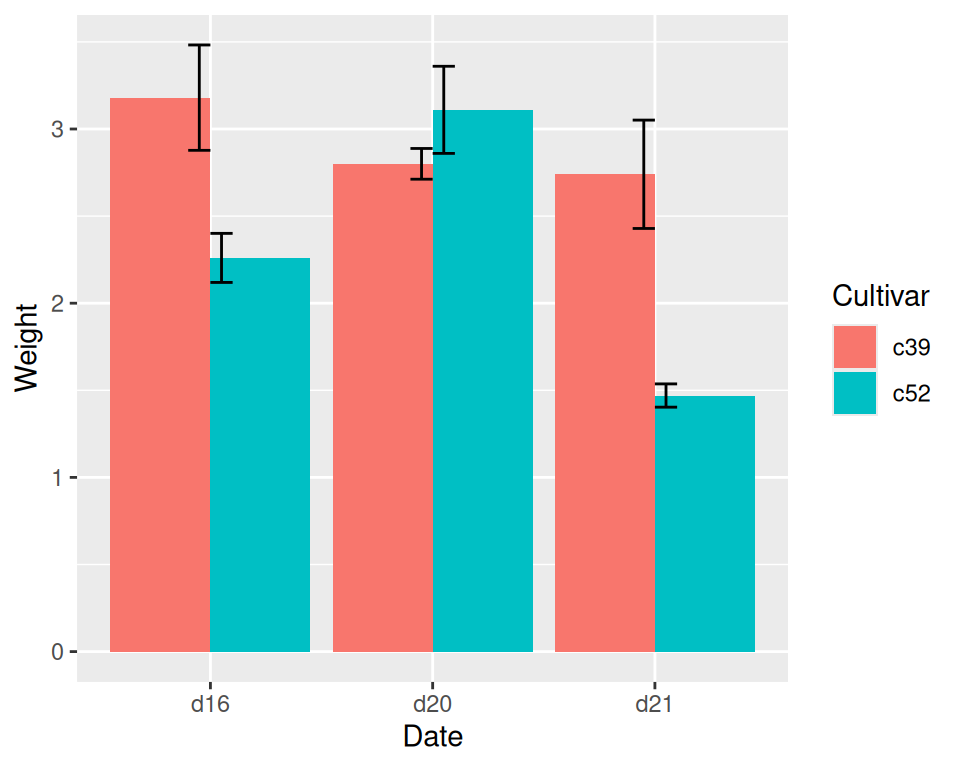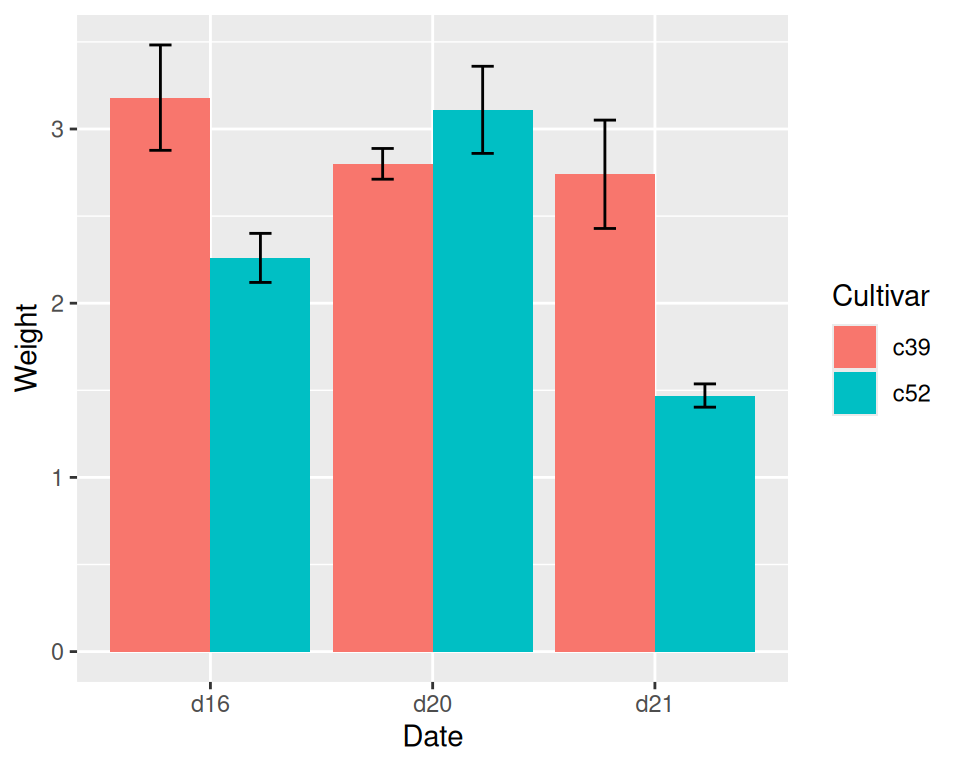Figure 7.15: error bars on a grouped bar graph without dodging width specified (left); with dodging width specified (right)

Note

Notice that we used `position = "dodge"`, which is shorthand for `position = position_dodge()`, in the first version. But to pass a specific value, we have to spell it out, as in `position_dodge(0.9)`.

For line graphs, if the error bars are a different color than the lines and points, you should draw the error bars first, so that they are underneath the points and lines. Otherwise the error bars will be drawn on top of the points and lines, which won’t look right.

Additionally, you should dodge all the geometric elements so that they will align with the error bars, as shown in Figure 7.16:

``````pd <- position_dodge(.3)  # Save the dodge spec because we use it repeatedly

ggplot(cabbage_exp, aes(x = Date, y = Weight, colour = Cultivar, group = Cultivar)) +
geom_errorbar(
aes(ymin = Weight - se, ymax = Weight + se),
width = .2,
size = 0.25,
colour = "black",
position = pd
) +
geom_line(position = pd) +
geom_point(position = pd, size = 2.5)

# Thinner error bar lines with size = 0.25, and larger points with size = 2.5``````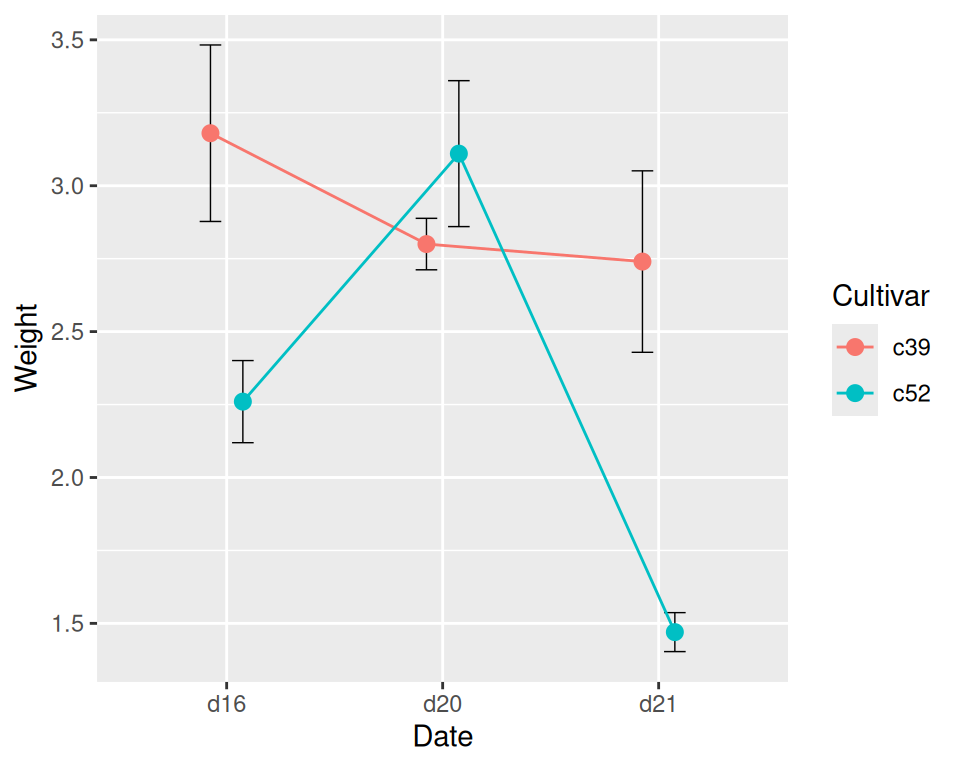Figure 7.16: Error bars on a line graph, dodged so they don’t overlap

Notice that we set `colour = "black"` to make the error bars black; otherwise, they would inherit `colour`. We also made sure the `Cultivar` was used as a grouping variable by mapping it to group.

When a discrete variable is mapped to an aesthetic like colour or fill (as in the case of the bars), that variable is used for grouping the data. But by setting the colour of the error bars, we made it so that the variable for colour was not used for grouping, and we needed some other way to inform ggplot that the two data entries at each x were in different groups so that they would be dodged.

See Recipe 3.2 for more about creating grouped bar graphs, and Recipe 4.3 for more about creating line graphs with multiple lines.

See Recipe 15.18 for calculating summaries with means, standard deviations, standard errors, and confidence intervals.

See Recipe 4.9 for adding a confidence region when the data has a higher density along the x-axis.

## 7.8 Adding Annotations to Individual Facets

### 7.8.1 Problem

You want to add annotations to each facet in a plot.

### 7.8.2 Solution

Create a new data frame with the faceting variable(s), and a value to use in each facet. Then use `geom_text()` with the new data frame (Figure 7.17):

``````# Create the base plot
mpg_plot <- ggplot(mpg, aes(x = displ, y = hwy)) +
geom_point() +
facet_grid(. ~ drv)

# A data frame with labels for each facet
f_labels <- data.frame(drv = c("4", "f", "r"), label = c("4wd", "Front", "Rear"))

mpg_plot +
geom_text(x = 6, y = 40, aes(label = label), data = f_labels)

# If you use annotate(), the label will appear in all facets
mpg_plot +
annotate("text", x = 6, y = 42, label = "label text")``````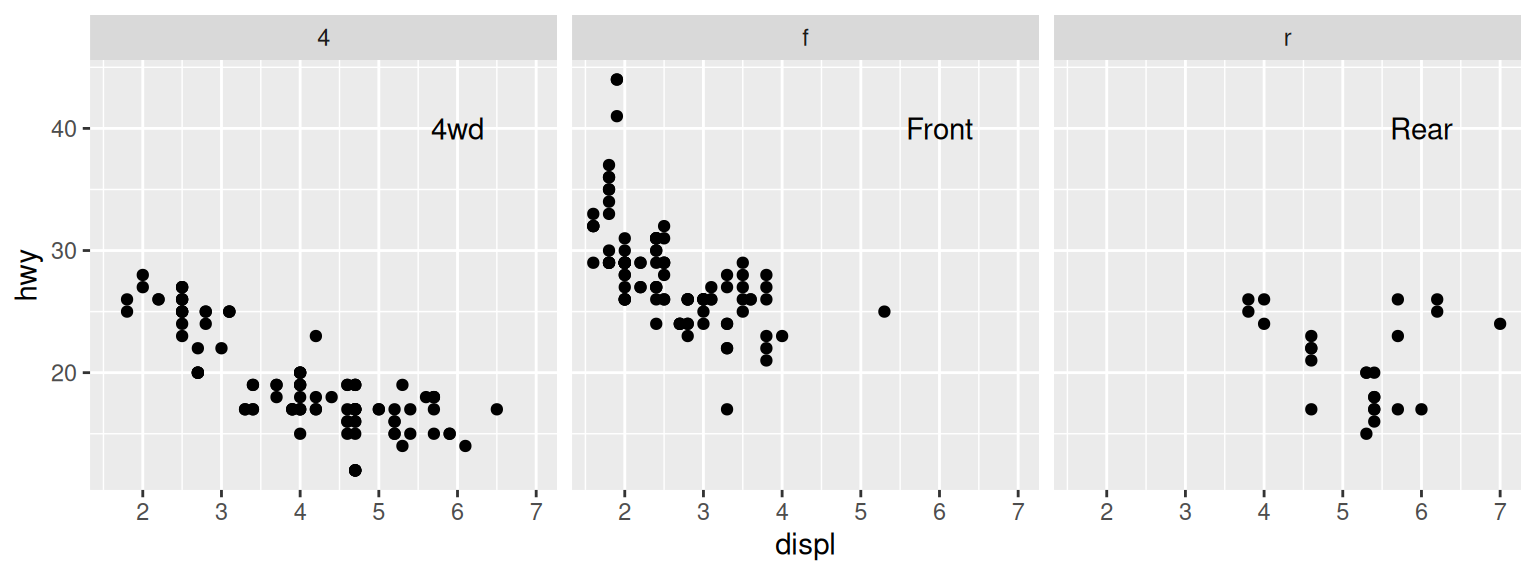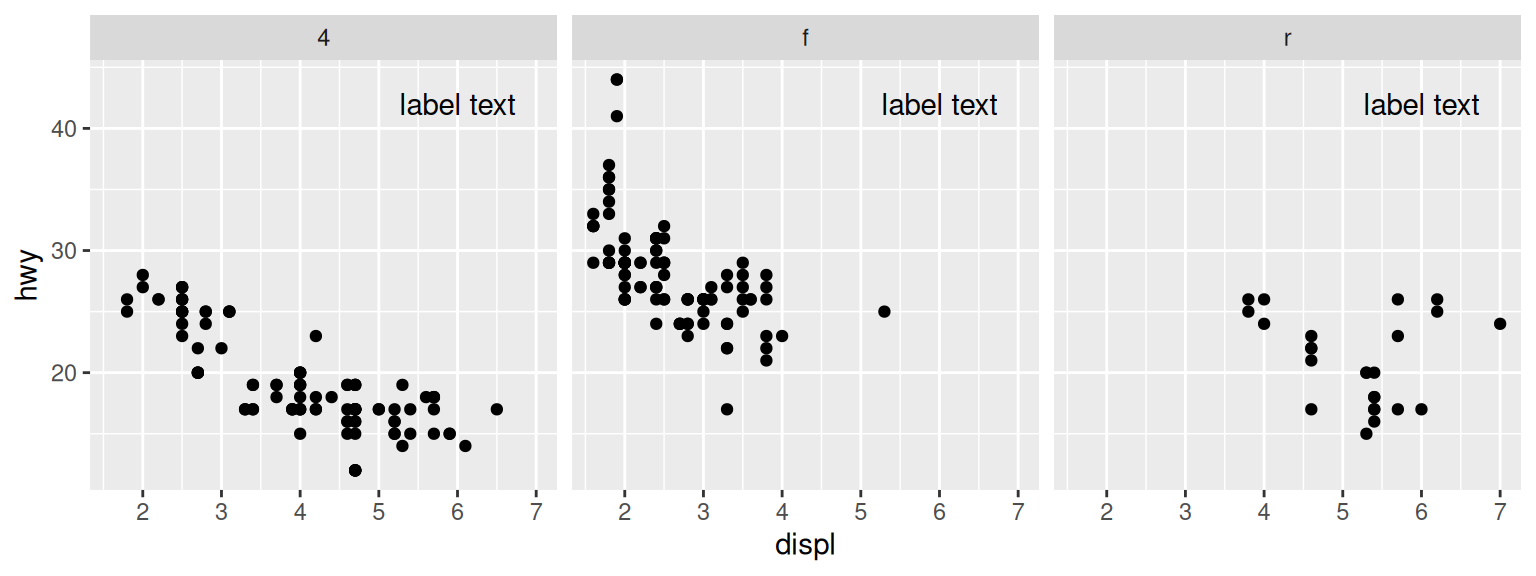Figure 7.17: Top: different annotations in each facet; bottom: the same annotation in each facet

### 7.8.3 Discussion

This method can be used to display information about the data in each facet, as shown in Figure 7.18. For example, in each facet we can show linear regression lines, the formula for each line, and the r2 value. To do this, we’ll write a function that takes a data frame and returns another data frame containing a string for a regression equation, and a string for the r2 value. Then we’ll use dplyr’s `do()` function to apply that function to each group of the data:

``````# This function returns a data frame with strings representing the regression
# equation, and the r^2 value.
# These strings will be treated as R math expressions
lm_labels <- function(dat) {
mod <- lm(hwy ~ displ, data = dat)
formula <- sprintf("italic(y) == %.2f %+.2f * italic(x)",
round(coef(mod), 2), round(coef(mod), 2))
r <- cor(dat\$displ, dat\$hwy)
r2 <- sprintf("italic(R^2) == %.2f", r^2)
data.frame(formula = formula, r2 = r2, stringsAsFactors = FALSE)
}

library(dplyr)
labels <- mpg %>%
group_by(drv) %>%
do(lm_labels(.))

labels
#> # A tibble: 3 x 3
#> # Groups:   drv 
#>   drv   formula                              r2
#>   <chr> <chr>                                <chr>
#> 1 4     italic(y) == 30.68 -2.88 * italic(x) italic(R^2) == 0.65
#> 2 f     italic(y) == 37.38 -3.60 * italic(x) italic(R^2) == 0.36
#> 3 r     italic(y) == 25.78 -0.92 * italic(x) italic(R^2) == 0.04

# Plot with formula and R^2 values
mpg_plot +
geom_smooth(method = lm, se = FALSE) +
geom_text(data = labels, aes(label = formula), x = 3, y = 40, parse = TRUE, hjust = 0) +
geom_text(x = 3, y = 35, aes(label = r2), data = labels, parse = TRUE, hjust = 0)``````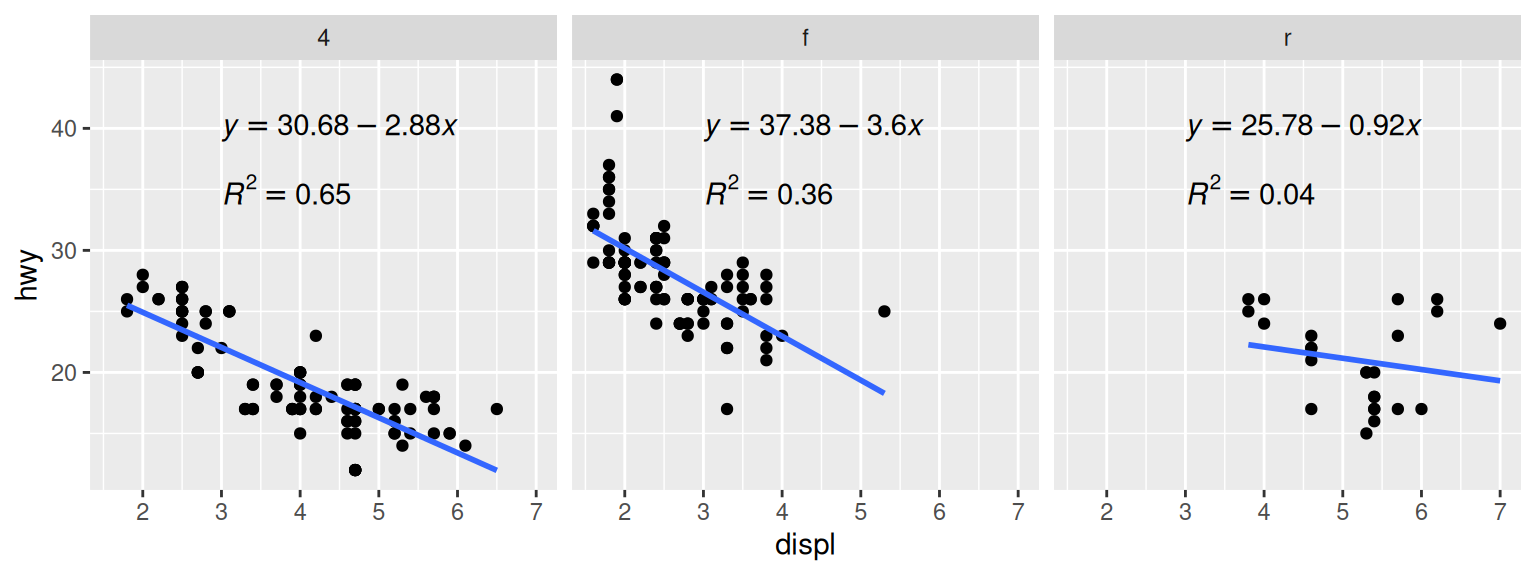Figure 7.18: Annotations in each facet with information about the data

We needed to write our own function here because generating the linear model and extracting the coefficients requires operating on each subset data frame directly. If you just want to display the r2 values, it’s possible to do something simpler, by using the `group_by()` and with the `summarise()` function and then passing additional arguments for `summarise()`:

``````# Find r^2 values for each group
labels <- mpg %>%
group_by(drv) %>%
summarise(r2 = cor(displ, hwy)^2)

labels\$r2 <- sprintf("italic(R^2) == %.2f", labels\$r2)
labels
#> # A tibble: 3 x 2
#>   drv   r2
#>   <chr> <chr>
#> 1 4     italic(R^2) == 0.65
#> 2 f     italic(R^2) == 0.36
#> 3 r     italic(R^2) == 0.04``````

Text geoms aren’t the only kind that can be added individually for each facet. Any geom can be used, as long as the input data is structured correctly.

If you want to make prediction lines from your own model objects, instead of having ggplot2 do it for you with `stat_smooth()`, see Recipe 5.8.